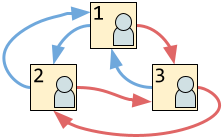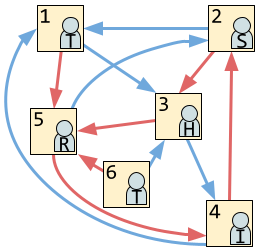시간 제한메모리 제한제출정답맞힌 사람정답 비율
서브태스크 참고 (추가 시간 없음) 1024 MB876100.000%

## 문제

The Google Coding Competitions team is setting up a new theme park. As in any good theme park, we want to have actors dressed up as mascots to interact with visitors. Because we are in a rush to open, we decided to use the letters from CODE JAM, KICK START, and HASH CODE as mascots, for a total of $13$ different mascots (the letters ACDEHIJKMORST).

The park's only attraction is a maze that has a set of $\mathbf{N}$ rooms numbered from $1$ to $\mathbf{N}$. Each room has a left exit and a right exit. Each exit takes the visitor to another room. Exits cannot be used in reverse; for example, if room $2$ has an exit to room $3$, you cannot go back from room $3$ to room $2$ unless room $3$ also happens to have an exit to room $2$.We want to place exactly one of our $13$ mascots in each room. Each letter may be present in zero, one, or more rooms of the maze. To increase variety, we want to place mascots so that any three (not necessarily distinct) rooms that a visitor can visit consecutively have three different mascots.

Can you help us choose a mascot for each room such that this goal is met, or let us know that it cannot be done?

## 입력

The first line of the input gives the number of test cases, $\mathbf{T}$. $\mathbf{T}$ test cases follow. Each test case consists of $3$ lines. The first line contains a single integer $\mathbf{N}$, representing the number of rooms in the maze. The second line contains $\mathbf{N}$ integers $\mathbf{L_1}, \mathbf{L_2}, \dots, \mathbf{L_N}$, representing that the left exit from room $i$ leads to room $\mathbf{L_i}$. The third and last line contains $\mathbf{N}$ integers $\mathbf{R_1}, \mathbf{R_2}, \dots, \mathbf{R_N}$, representing that the right exit from room $i$ leads to room $\mathbf{R_i}$.

## 출력

For each test case, output one line containing Case #x: y, where $x$ is the test case number (starting from 1) and $y$ is IMPOSSIBLE if there is no way to assign mascots while obeying the rules explained above. Otherwise, $y$ is an $\mathbf{N}$ character long string. The $i$-th character of $y$ should be an uppercase letter from the set ACDEHIJKMORST, representing that you wish to assign that mascot to the $i$-th room.

## 제한

• $1 \le \mathbf{T} \le 100$.
• $\mathbf{L_i} \neq i$, for all $i$. $\mathbf{R_i} \neq i$, for all $i$. $1 \le \mathbf{L_i} \lt \mathbf{R_i} \le \mathbf{N}$, for all $i$.

## Test Set 1 (12점)

시간 제한: 20 초

• $3 \le \mathbf{N} \le 100$.

## Test Set 2 (13점)

시간 제한: 45 초

• $3 \le \mathbf{N} \le 10^5$.

## 예제 입력 1

4
3
2 1 1
3 3 2
6
3 1 4 1 2 3
5 3 5 2 4 5
20
2 3 4 5 6 7 8 9 10 11 12 13 14 15 16 17 18 19 1 1
3 4 5 6 7 8 9 10 11 12 13 14 15 16 17 18 19 20 20 2
19
2 3 4 5 6 7 8 9 10 11 12 13 14 15 16 17 18 1 1
3 4 5 6 7 8 9 10 11 12 13 14 15 16 17 18 19 19 3


## 예제 출력 1

Case #1: IMPOSSIBLE
Case #2: TSHIRT
Case #3: HCJKSHCJKSHCJKSHCJKS
Case #4: CODEJAMROCKSTHEMOST


## 힌트

Sample Case #1 is the image in the problem statement. It is possible to visit rooms 1, 2, and 1 consecutively (which visits room 1 twice), so the case is impossible.

Sample Case #2 has the following layout (blue arrows represent the left exits and red arrows represent the right exits):One of many valid answers is to assign mascots as indicated. Notice that although we do not need to assign two T mascots in this case, we have done so in a way that does not break the rules.

Sample Cases #3 and #4 are possible, but require the use of multiple copies of some mascots.

## 채점 및 기타 정보

• 예제는 채점하지 않는다.